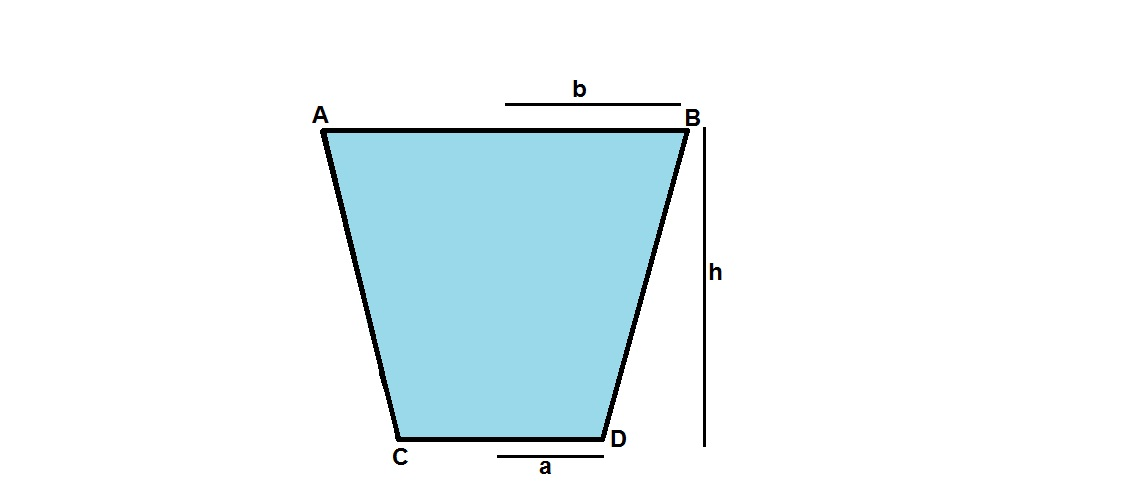# Balti Physics!!A bucket ( also known as "Balti" in Hindi ! ) is fully filled with water. The bucket has the shape of a symmetrical frustum of upper radius "b" and lower radius "a". It is of height "h". Find the net downward force (in SI Units) that the water exerts on the sides of the bucket i.e, sides AC and BD. Neglect atmospheric pressure.

$\textbf{NOTE -}$

$\textbf{a= 0.5 m}$

$\textbf{b= 1 m}$

$\textbf{h}$ = $\frac{3}{π} m$

$g = 10 m/s^{2}$

Density of water = $10^{3} kg/m^{3}$

×